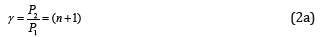• Submissions# Systems of Conformational Processes

Korablev GA*

Doctor of Chemical Sciences, Russia

*Corresponding author:Korablev GA, Doctor of Chemical Sciences, Professor, Izhevsk State Agricultural Academy, Russia

Submission: March 25, 2019;Published: April 01, 2019

DOI: 10.31031/RDMS.2019.10.000736ISSN: 2576-8840
Volume10 Issue3

#### Opinion

Isomorphism as a phenomenon is usually considered as applicable to crystal structures. But, obviously, similar processes can also flow between molecular compounds, where the bond energy can be evaluated via the relative difference of electron densities of valence orbitals of interacting atoms . Since Р-parameter possesses wave properties (similar to Ψ’-function), mainly the regularities in the interference of the corresponding waves should be fulfilled.

Oscillation weakening (in anti-phase) takes place if the difference in wave move (Δ) equals the odd number of semi-waves, it is interference minimum:The difference in wave move (Δ) for Р-parameters can be evaluated via their relative valueOscillation enhancing (in phase) takes place if the difference in wave move equals an even number of semi-waves, it is interference maximum:As applicable to P-parameters, the maximum enhance of interaction in the phase corresponds to the interactions of similarly charged systems or systems homogeneous by their properties and functions (e.g. between the fragments or blocks of complex organic structures, such as CH2 and NNO2).

And then:It was shown  that the molecular negativity is numerically equal to the P-parameter of the first valence electron divided by 3. Hydrogen atom, element No 1 with orbital 1S1 defines the main energy criteria of structural interactions (their “ancestor”).

Table 1 shows its three PE-parameters corresponding to three different characteristics of the atom. R1=0.5292А0-arbital radius-quantum-mechanical characteristic gives the initial main value of PE-parameter equaled to 9.0644eV;

R2=0.375 А0- distance equaled to the half of the bond energy in Н2 molecule. But if hydrogen atom is bound with other atoms, its covalent radius is ≈ 0.28А0. It was shown earlier 5 that the condition for the formation of stable structures is an approximate equality of the P-parameter of the subsystems. Relative parameter difference is estimated by alpha factor.

In accordance with equation (2) P2=P1(n+1), therefore P1 ≈ 9.0644eV, P2 ≈ 18.129eV. These are the values of possible energy criteria of stable (stationary) structures. The dimensional characteristic 0.375 А° does not satisfy them, therefore, there is a transition onto to the covalence radius ≈ 0.28А°, which provides the value of P-parameter approximately equaled to P2.

It was shown earlier that the condition for the formation of stable structures is an approximate equality of the P-parameter of the subsystems. From a big number of different combinations of interactions, we can obtain series with approximately equal values of P-parameters of atoms (or radicals). Such series, by initial values of hydrogen atom, are given in Table 1 (at α < 7.5%).

Table 1:Energy parameters of atoms and radicals.First series for PE=9.0644eV-the main, initial, where Н,С, О, N basic bio structural atoms have PE-parameters only of the first electron and interactions proceed in the phase. Second series for Р’E =12.792eV is the non-rational, pathological as it more corresponds to the interactions in anti-phase: by equation (1а) Р’’E =13.596 eV. Coefficient α between the parameters Р’E and Р’’E equals 6.1%, thus defining the possibility of forming “false” biostructures containing the molecular hydrogen Н2. Coefficient α between series I and II is 34.1%, thus confirming the irrationality of series II.

Third series for Р’E=17.132eV- stationary as the interactions are in the phase: by equation (1а) Р’’E =18.129 eV (α =5.5%). Specific local energy effects (electromagnetic fields, radiation, etc.) upon structural conformations can increasingly follow the pathological series II. Isn’t it one of the reasons of normal functioning failure in biosystems, e.g., in oncological diseases? If so, some practical recommendations can be done, which come down to the necessity of converting the molecular hydrogen into the atomic one, e.g., through the interaction with hydroxyl OH group.

From Table 1 it is seen that the majority of atoms and radicals, depending on the bond types and bond lengths, have PE-parameters of different series. When introducing the stem cells, it is important for the molecular hydrogen not to be present in their structures. Otherwise atoms and radicals can transfer into the series II and disturb the vital functions of the main first system. The reverse method can be applied to utilize polymeric materials transferring their molecular fragments into the pathological series II.

#### Conclusion

1. Р-parameters of the first valence electron of atoms define the energy characteristics of stationary states (in normal state) under the condition of the maximum of wave processes.

Under the condition of the minimum of such interactions, the pathological (but not stationary) biostructures containing

We at Crimson Publishing are a group of people with a combined passion for science and research, who wants to bring to the world a unified platform where all scientific know-how is available read more...